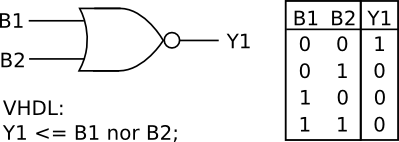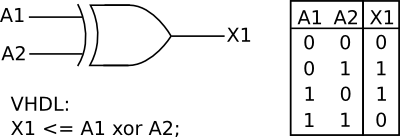Starting Electronics needs your help! Please make a donation to help cover our hosting and other costs. Click the donate button to send a donation of any amount.# Tutorial 3: NAND, NOR, XOR and XNOR Gates in VHDL

Created on: 12 December 2012

In the previous tutorial, we looked at AND gates, OR gates and signals in VHDL. This tutorial covers the remaining gates, namely NAND, NOR, XOR and XNOR gates in VHDL.

## NAND and NOR Logic Gates in VHDL

### NAND Gate

The VHDL nand keyword is used to create a NAND gate:NAND Gate with Truth Table and VHDL

### NOR Gate

The VHDL nor keyword is used to create a NOR gate:NOR Gate with Truth Table and VHDL

### NAND and NOR VHDL Project

This code listing shows the NAND and NOR gates implemented in the same VHDL code. Two separate gates are created that each have two inputs.

```library IEEE;
use IEEE.STD_LOGIC_1164.ALL;

entity nand_nor_top is
Port ( A1 : in  STD_LOGIC;      -- NAND gate input 1
A2 : in  STD_LOGIC;      -- NAND gate input 2
X1 : out  STD_LOGIC;     -- NAND gate output
B1 : in  STD_LOGIC;      -- NOR gate input 1
B2 : in  STD_LOGIC;      -- NOR gate input 2
Y1 : out  STD_LOGIC);    -- NOR gate output
end nand_nor_top;

architecture Behavioral of nand_nor_top is
begin
X1 <= A1 nand A2;    -- 2 input NAND gate
Y1 <= B1 nor B2;     -- 2 input NOR gate
end Behavioral;
```

The code listing below shows the same code as above, but with compensation for the inverting inputs on the home made Xilinx CPLD board. The need for compensation is explained in tutorial 2 AND Gates, OR Gates and Signals in VHDL.

```library IEEE;
use IEEE.STD_LOGIC_1164.ALL;

entity nand_nor_top is
Port ( IN1 : in  STD_LOGIC;
IN2 : in  STD_LOGIC;
IN3 : in  STD_LOGIC;
IN4 : in  STD_LOGIC;
OUT1 : out  STD_LOGIC;
OUT2 : out  STD_LOGIC);
end nand_nor_top;

architecture Behavioral of nand_nor_top is
signal A1 : STD_LOGIC;
signal A2 : STD_LOGIC;
signal X1 : STD_LOGIC;
signal B1 : STD_LOGIC;
signal B2 : STD_LOGIC;
signal Y1 : STD_LOGIC;
begin

X1 <= A1 nand A2;
Y1 <= B1 nor B2;

-- compensation for inverting inputs and outputs
A1   <= not IN1;
A2   <= not IN2;
OUT1 <= not X1;
B1   <= not IN3;
B2   <= not IN4;
OUT2 <= not Y1;

end Behavioral;
```

Download nand_nor.zip (5.9kB) which contains the VHD, UCF and JED files for the NAND and NOR gates. The JED file is for configuring the home made CPLD board.

This video shows the NAND gate and then the NOR gate implemented on the home made CPLD board.

Can't see the video? View on YouTube →

Books that may interest you:## Exclusive-OR and Exclusive-NOR Logic Gates in VHDL

### XOR Gate

The VHDL xor keyword is used to create an XOR gate:XOR Gate with Truth Table and VHDL

### XNOR Gate

The VHDL xnor keyword is used to create an XNOR gate:XNOR Gate with Truth Table and VHDL

### XOR and XNOR VHDL Project

This listing shows an XOR and XNOR gate in VHDL.

```library IEEE;
use IEEE.STD_LOGIC_1164.ALL;

entity xor_xnor_top is
Port ( A1 : in  STD_LOGIC;      -- XOR gate input 1
A2 : in  STD_LOGIC;      -- XOR gate input 2
X1 : out  STD_LOGIC;     -- XOR gate output
B1 : in  STD_LOGIC;      -- XNOR gate input 1
B2 : in  STD_LOGIC;      -- XNOR gate input 2
Y1 : out  STD_LOGIC);    -- XNOR gate output
end xor_xnor_top;

architecture Behavioral of xor_xnor_top is
begin
X1 <= A1 xor A2;		-- 2 input exclusive-OR gate
Y1 <= B1 xnor B2;		-- 2 input exclusive-NOR gate
end Behavioral;
```

The VHDL code listing below shows the same code as above, but with compensation for inverting inputs and outputs on the board.

```library IEEE;
use IEEE.STD_LOGIC_1164.ALL;

entity xor_xnor_top is
Port ( IN1 : in  STD_LOGIC;
IN2 : in  STD_LOGIC;
IN3 : in  STD_LOGIC;
IN4 : in  STD_LOGIC;
OUT1 : out  STD_LOGIC;
OUT2 : out  STD_LOGIC);
end xor_xnor_top;

architecture Behavioral of xor_xnor_top is
signal A1 : STD_LOGIC;
signal A2 : STD_LOGIC;
signal X1 : STD_LOGIC;
signal B1 : STD_LOGIC;
signal B2 : STD_LOGIC;
signal Y1 : STD_LOGIC;
begin

X1 <= A1 xor A2;

Y1 <= B1 xnor B2;

-- compensation for inverting inputs and outputs
A1   <= not IN1;
A2   <= not IN2;
OUT1 <= not X1;
B1   <= not IN3;
B2   <= not IN4;
OUT2 <= not Y1;

end Behavioral;
```

Download xor_xnor.zip (5.9kB) which contains the VHD, UCF and JED files for the XOR and XNOR gates. The JED file is for configuring the home made CPLD board.

This video shows the XOR gate and then the XNOR gate on the home made CPLD board.

Can't see the video? View on YouTube →